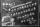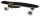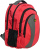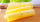# Calculator expression with brackets

The calculator performs basic and advanced operations with fractions, expressions with fractions combined with integers, decimals, and mixed numbers. It also shows detailed step-by-step information about the fraction calculation procedure. Solve problems with two, three, or more fractions and numbers in one expression.

### 1/3 * (1/2 - 33/8) = -23/24 ≅ -0.9583333

Spelled result in words is minus twenty-three twenty-fourths.

### How do you solve fractions step by step?

1. Conversion a mixed number 3 3/8 to a improper fraction: 3 3/8 = 3 3/8 = 3 · 8 + 3/8 = 24 + 3/8 = 27/8

To find new numerator:
a) Multiply the whole number 3 by the denominator 8. Whole number 3 equally 3 * 8/8 = 24/8
b) Add the answer from previous step 24 to the numerator 3. New numerator is 24 + 3 = 27
c) Write a previous answer (new numerator 27) over the denominator 8.

Three and three eighths is twenty-seven eighths
2. Subtract: 1/2 - 27/8 = 1 · 4/2 · 4 - 27/8 = 4/8 - 27/8 = 4 - 27/8 = -23/8
For adding, subtracting, and comparing fractions, it is suitable to adjust both fractions to a common (equal, identical) denominator. The common denominator you can calculate as the least common multiple of both denominators - LCM(2, 8) = 8. In practice, it is enough to find the common denominator (not necessarily the lowest) by multiplying the denominators: 2 × 8 = 16. In the next intermediate step, the fraction result cannot be further simplified by canceling.
In words - one half minus twenty-seven eighths = minus twenty-three eighths.
3. Multiple: 1/3 * the result of step No. 2 = 1/3 * (-23/8) = 1 · (-23)/3 · 8 = -23/24
Multiply both numerators and denominators. Result fraction keep to lowest possible denominator GCD(-23, 24) = 1. In the next intermediate step, the fraction result cannot be further simplified by canceling.
In words - one third multiplied by minus twenty-three eighths = minus twenty-three twenty-fourths.

#### Rules for expressions with fractions:

Fractions - use the slash “/” between the numerator and denominator, i.e., for five-hundredths, enter 5/100. If you are using mixed numbers, be sure to leave a single space between the whole and fraction part.
The slash separates the numerator (number above a fraction line) and denominator (number below).

Mixed numerals (mixed fractions or mixed numbers) write as non-zero integer separated by one space and fraction i.e., 1 2/3 (having the same sign). An example of a negative mixed fraction: -5 1/2.
Because slash is both signs for fraction line and division, we recommended use colon (:) as the operator of division fractions i.e., 1/2 : 3.

Decimals (decimal numbers) enter with a decimal point . and they are automatically converted to fractions - i.e. 1.45.

The colon : and slash / is the symbol of division. Can be used to divide mixed numbers 1 2/3 : 4 3/8 or can be used for write complex fractions i.e. 1/2 : 1/3.
An asterisk * or × is the symbol for multiplication.
Plus + is addition, minus sign - is subtraction and ()[] is mathematical parentheses.
The exponentiation/power symbol is ^ - for example: (7/8-4/5)^2 = (7/8-4/5)2

The calculator follows well-known rules for order of operations. The most common mnemonics for remembering this order of operations are:
PEMDAS - Parentheses, Exponents, Multiplication, Division, Addition, Subtraction.
BEDMAS - Brackets, Exponents, Division, Multiplication, Addition, Subtraction
BODMAS - Brackets, Of or Order, Division, Multiplication, Addition, Subtraction.
GEMDAS - Grouping Symbols - brackets (){}, Exponents, Multiplication, Division, Addition, Subtraction.
Be careful, always do multiplication and division before addition and subtraction. Some operators (+ and -) and (* and /) has the same priority and then must evaluate from left to right.

## Fractions in word problems:

• There 12There are 42 students in the class and 2/3 of them are girls. How may girls are there in the class?
• Paper clipsMrs. Bright is organizing her office supplies. There are 5 open boxes of paper clips in her desk drawer. Each box has 1/2 of the paper clips remaining. How many boxes of paper clips are left?
• Scouts 44/7 of the students in a school are boys. If 3/8 of the boys are scouts, how many scouts are there in a school of 1878 students?
• A storeA store received a shipment of 240 skateboards. In three weeks, it sold 1/3 of those skateboards. How many skateboards did the store sell?
• KellyKelly and Dan are collecting clothes for a clothing drive. Dan collected 1/2 as many clothes as Kelly did. If Kelly collected 3 bags of clothes, how many bags of clothes did Dan collect?
• Slices of pizzaMaria ate 1/4 of a pizza. If there were 20 slices of pizza, how many slices did Maria eat?
• One-third 2One-third of the people in a barangay petitioned the council to allow them to plant in vacant lots, and another 1/5 of the people petitioned to have a regular garbage collection. What FRACTION of the barangay population made the petition?
• A shopkeeperA shopkeeper cuts a wheel of cheese into 10 equal wedges. A customer buys one-fifth of the wheel. How many wedges does the customer buy? Use the number line to help find the solution.
• SarahSarah has a photography blog. 3/7 of her photos are of nature, 1/4 of the rest are of her friends. What fraction of all of Sarah's photos is of her friends?
• RickyRicky painted 3/5 of the side of the garage. When he repainted ½ of this part, what part of the side of the garage did he painted twice?
• Farmer PeterFarmer Peter paints 12 chicken coop. He started painting this day morning. Now he only has 1/4 of the chicken coop left to paint this afternoon. How many chicken coops did farmer Peter paint this morning?
• Fraction of a fractionWhat is two-quarters of twelve?
• Equivalent fractionsAre these two fractions equivalent -4/9 and 11/15?